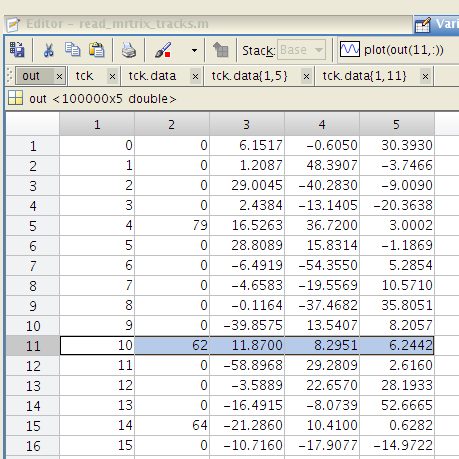# Tckgen -output_seeds

Hi everyone

Just a simple question,when I use the option -output_seeds,I don’t know the meaning of Seed_index
For example :the (11,2) is 62 in the picture ,then I use the command read_mrtrix_tracks to see the tck.data{1,11},I find that the Pos_x,y,z are same in the 63So I want to know the reason why saving 62 in the out_put_seeds file. Does it mean that the Pos_x,y,z in the second picture is the true track starting ? or others ?

Thanks

Hi,

The `Seed_index` field is the index of the streamline point that was used as the streamline seed. This should be preserved even in the presence of downsampling when running `tckgen`.

The reason for the discrepancy here is that MRtrix3 code (and C++ code universally) uses 0 as the starting index of an array, whereas MatLab uses 1. So for instance, for all the streamlines where the indicated seed index is 0 (the first point on the streamline), this will in fact appear on row number 1 in MatLab. Therefore if you are examining the track data in MatLab, you will need to add 1 to every streamline seed index reported by the `-output_seeds` option in order to find the corresponding data row.

Cheers
Rob

Thanks,I want to make sure that the 63 is the streamline starting point,so the tck.data{1,11} length of this streamline is not 72 but 72 minus 63 .Is right?

If bidirectional tracking is performed, then the first point of the track is not guaranteed to be the point from which the streamline was seeded; this is precisely why the seed index field is provided. Combining the total streamline length (as evident in the raw track data), with knowledge of the seed point index, would allow you to know how far each streamline propagated from the seed in either direction. Note that the track data before the seed point corresponds to the first tracking propagation in the case of bidirectional tracking.

Thanks very much,I get it ! Because I use the tckgen -act -seed_gmwmi , I think the tracking all is unidirectional ,the seed starting point is the first of the tck.data{}.But I ignored that tracking at the boundary of white matter and sub-cortical grey matter is bidirectional